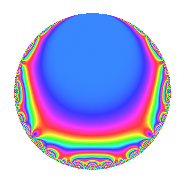# Properties

 Label 4014.2.a.lLevel 4014 Weight 2 Character orbit 4014.a Self dual Yes Analytic conductor 32.052 Analytic rank 0 Dimension 2 CM No Inner twists 1

# Related objects

## Newspace parameters

 Level: $$N$$ = $$4014 = 2 \cdot 3^{2} \cdot 223$$ Weight: $$k$$ = $$2$$ Character orbit: $$[\chi]$$ = 4014.a (trivial)

## Newform invariants

 Self dual: Yes Analytic conductor: $$32.0519513713$$ Analytic rank: $$0$$ Dimension: $$2$$ Coefficient field: $$\Q(\sqrt{13})$$ Coefficient ring: $$\Z[a_1, \ldots, a_{11}]$$ Coefficient ring index: $$1$$ Fricke sign: $$-1$$ Sato-Tate group: $\mathrm{SU}(2)$

## $q$-expansion

Coefficients of the $$q$$-expansion are expressed in terms of $$\beta = \frac{1}{2}(1 + \sqrt{13})$$. We also show the integral $$q$$-expansion of the trace form.

 $$f(q)$$ $$=$$ $$q + q^{2} + q^{4} -2 \beta q^{7} + q^{8} +O(q^{10})$$ $$q + q^{2} + q^{4} -2 \beta q^{7} + q^{8} + ( 5 - \beta ) q^{11} + ( 4 - \beta ) q^{13} -2 \beta q^{14} + q^{16} + ( 2 - 2 \beta ) q^{17} + ( 2 + \beta ) q^{19} + ( 5 - \beta ) q^{22} -4 q^{23} -5 q^{25} + ( 4 - \beta ) q^{26} -2 \beta q^{28} + ( 3 + \beta ) q^{29} + ( 2 + 2 \beta ) q^{31} + q^{32} + ( 2 - 2 \beta ) q^{34} + 2 \beta q^{37} + ( 2 + \beta ) q^{38} -4 q^{41} + ( -3 + 3 \beta ) q^{43} + ( 5 - \beta ) q^{44} -4 q^{46} + ( 3 - \beta ) q^{47} + ( 5 + 4 \beta ) q^{49} -5 q^{50} + ( 4 - \beta ) q^{52} + 3 \beta q^{53} -2 \beta q^{56} + ( 3 + \beta ) q^{58} + ( -2 + \beta ) q^{59} + ( -5 + \beta ) q^{61} + ( 2 + 2 \beta ) q^{62} + q^{64} + 2 \beta q^{67} + ( 2 - 2 \beta ) q^{68} + ( 2 + 4 \beta ) q^{71} + ( -2 - 3 \beta ) q^{73} + 2 \beta q^{74} + ( 2 + \beta ) q^{76} + ( 6 - 8 \beta ) q^{77} + ( -3 + 5 \beta ) q^{79} -4 q^{82} + ( 4 - 4 \beta ) q^{83} + ( -3 + 3 \beta ) q^{86} + ( 5 - \beta ) q^{88} + ( 8 + 4 \beta ) q^{89} + ( 6 - 6 \beta ) q^{91} -4 q^{92} + ( 3 - \beta ) q^{94} + ( -10 + 4 \beta ) q^{97} + ( 5 + 4 \beta ) q^{98} +O(q^{100})$$ $$\operatorname{Tr}(f)(q)$$ $$=$$ $$2q + 2q^{2} + 2q^{4} - 2q^{7} + 2q^{8} + O(q^{10})$$ $$2q + 2q^{2} + 2q^{4} - 2q^{7} + 2q^{8} + 9q^{11} + 7q^{13} - 2q^{14} + 2q^{16} + 2q^{17} + 5q^{19} + 9q^{22} - 8q^{23} - 10q^{25} + 7q^{26} - 2q^{28} + 7q^{29} + 6q^{31} + 2q^{32} + 2q^{34} + 2q^{37} + 5q^{38} - 8q^{41} - 3q^{43} + 9q^{44} - 8q^{46} + 5q^{47} + 14q^{49} - 10q^{50} + 7q^{52} + 3q^{53} - 2q^{56} + 7q^{58} - 3q^{59} - 9q^{61} + 6q^{62} + 2q^{64} + 2q^{67} + 2q^{68} + 8q^{71} - 7q^{73} + 2q^{74} + 5q^{76} + 4q^{77} - q^{79} - 8q^{82} + 4q^{83} - 3q^{86} + 9q^{88} + 20q^{89} + 6q^{91} - 8q^{92} + 5q^{94} - 16q^{97} + 14q^{98} + O(q^{100})$$

## Embeddings

For each embedding $$\iota_m$$ of the coefficient field, the values $$\iota_m(a_n)$$ are shown below.

For more information on an embedded modular form you can click on its label.

Label $$\iota_m(\nu)$$ $$a_{2}$$ $$a_{3}$$ $$a_{4}$$ $$a_{5}$$ $$a_{6}$$ $$a_{7}$$ $$a_{8}$$ $$a_{9}$$ $$a_{10}$$
1.1
 2.30278 −1.30278
1.00000 0 1.00000 0 0 −4.60555 1.00000 0 0
1.2 1.00000 0 1.00000 0 0 2.60555 1.00000 0 0
 $$n$$: e.g. 2-40 or 990-1000 Significant digits: Format: Complex embeddings Normalized embeddings Satake parameters Satake angles

## Inner twists

This newform does not admit any (nontrivial) inner twists.

## Atkin-Lehner signs

$$p$$ Sign
$$2$$ $$-1$$
$$3$$ $$1$$
$$223$$ $$1$$

## Hecke kernels

This newform can be constructed as the intersection of the kernels of the following linear operators acting on $$S_{2}^{\mathrm{new}}(\Gamma_0(4014))$$:

 $$T_{5}$$ $$T_{7}^{2} + 2 T_{7} - 12$$ $$T_{11}^{2} - 9 T_{11} + 17$$